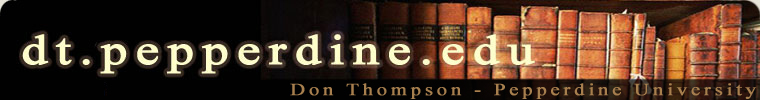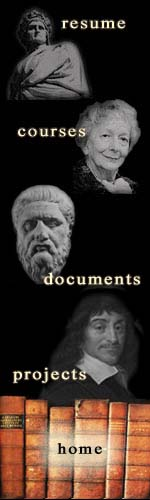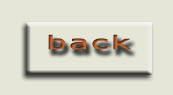Math 365 Chapter 2 Homework Due 2.17.20 1 4 6 9 13a more hints tomorrow information theory hw: no summary required, just 4 problems: 1. prove that Hmax occurs when pi = 1/n for all i. 2. do problem on bottom of p. 22 of raisbeck 3. suppose we toss a fair pair of dice and then transmit the sum - find the total amount of information possible for that source. 4. choose two paragraphs from the reading (let me know which ones) and caclulate their amounts of information. assume that any character NOT found in the passage has probability 0 and therefore is not part of the information calculation. spaces, punctation and letters do count If, for example, the text is simply: This sentence has a little bit of information. we get 3.803226567 bits of information. character count